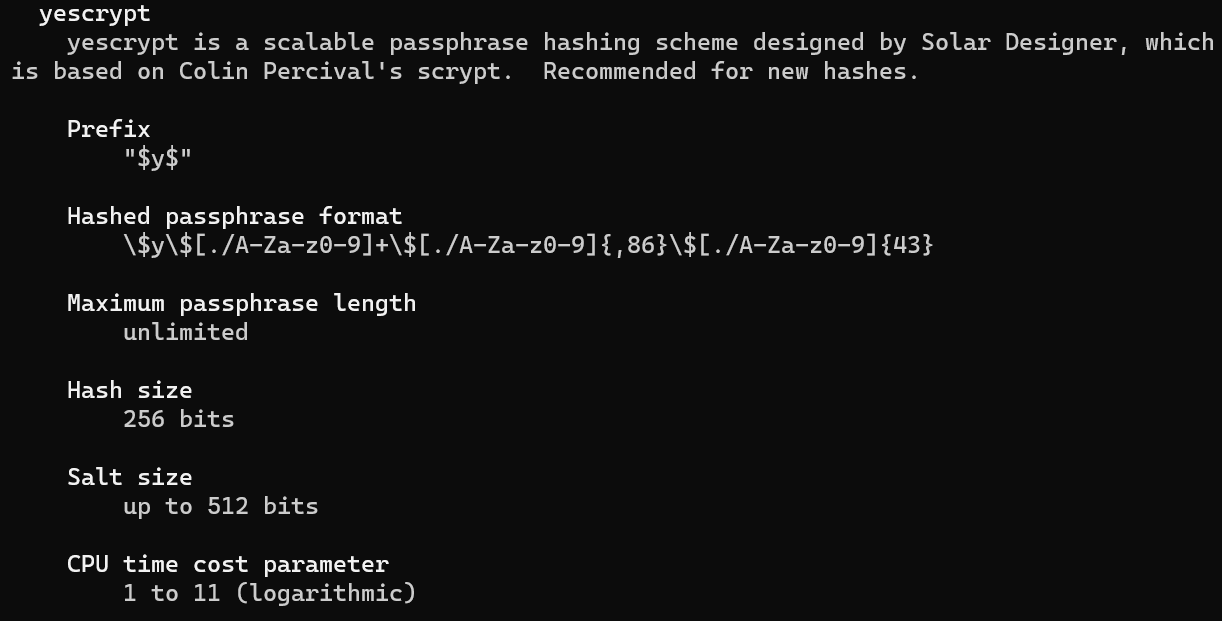View on GitHub

# Information Security

UUG Spring 2023

“The three golden rules to ensure computer security are: do not own a computer; do not power it on; and do not use it.” — Robert Morris

• Confidentiality
• Integrity
• Availability

## Confidentiality

• Assures that private or confidential information is not made available or disclosed to unauthorized individuals

## Integrity

• Assures that information and programs are changed only in a specified and authorized manner

## Availability

• Assures that systems work promptly and service is not denied to users

## Which Aspect of the CIA Triad is Most Important?

• Authenticity

• The property of being genuine and being able to be verified and trusted

• Confidence in the validity of a transmission, a message, or message originator
• Accountability

• The security goal that generates the requirement for actions of an entity to be traced uniquely to that entity.

• Non-repudiation

## Cryptography Concepts

• Plaintext

• The item we plan on encrypting. Human-readable.
• Key

• A secret.
• Ciphertext

• The output of encryption. Not human-readable.
• Cleartext

• The output of decryption (same as the input plaintext).

## Kerckhoffs’s principle

• Security must reside entirely in the secret key

• Assume all other information is known by the attacker

• This includes the:

• key-space (128-bit, 256-bit)
• algorithm (AES, RSA, ECC)
• hardware (Enigma)

## Types of Ciphers

• Block

• Message is partitioned into fixed-size blocks (typically 64 or 128-bits)

• DES, AES, RSA
• Stream

• Encrypts a digital data stream one bit or one byte at a time.

## Electronic Codebook (ECB)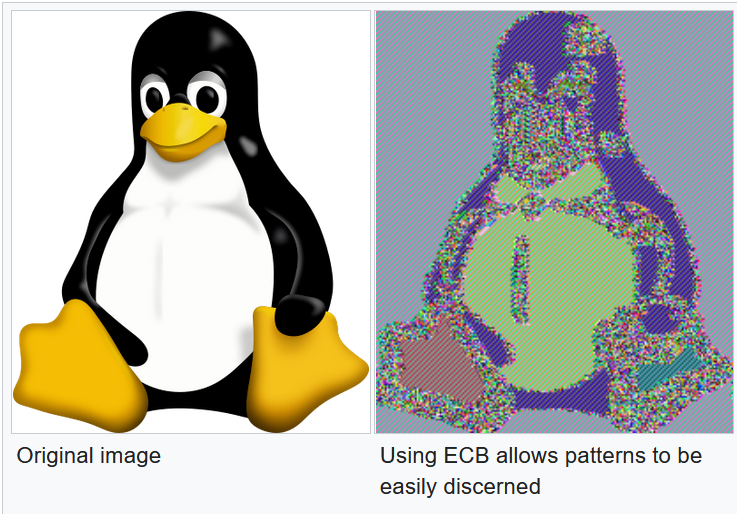## Cipher Block Chaining (CBC)

• Duplicate data is not reflected in ciphertext

• Malformed/incorrect blocks affect all following blocks## Output feedback (OFB)

• Can prepare encryption and decryption in advance

• Malformed/incorrect blocks affect only the current block## Types of Encryption

Symmetric Asymmetric
Fast Slow
Good for large data transfers Good for small data transfers
Only provides confidentiality Can provide confidentiality, authentication, and non-repudiation

## Symmetric Encryption## “Perfect” Encryption - One-Time Pad (OTP)

• Major rules of OTP:
• Key must be the same size as the message
• Key must be truly random
• Keys must never be reused## Feistel Cipher

• Created by German physicist Horst Feistel and patented the early 1970’s
• The Feistel Cipher is a design model (template) that is used to create different block ciphers.
• Blowfish, DES, RC5, Twofish

## How the Feistel Cipher Works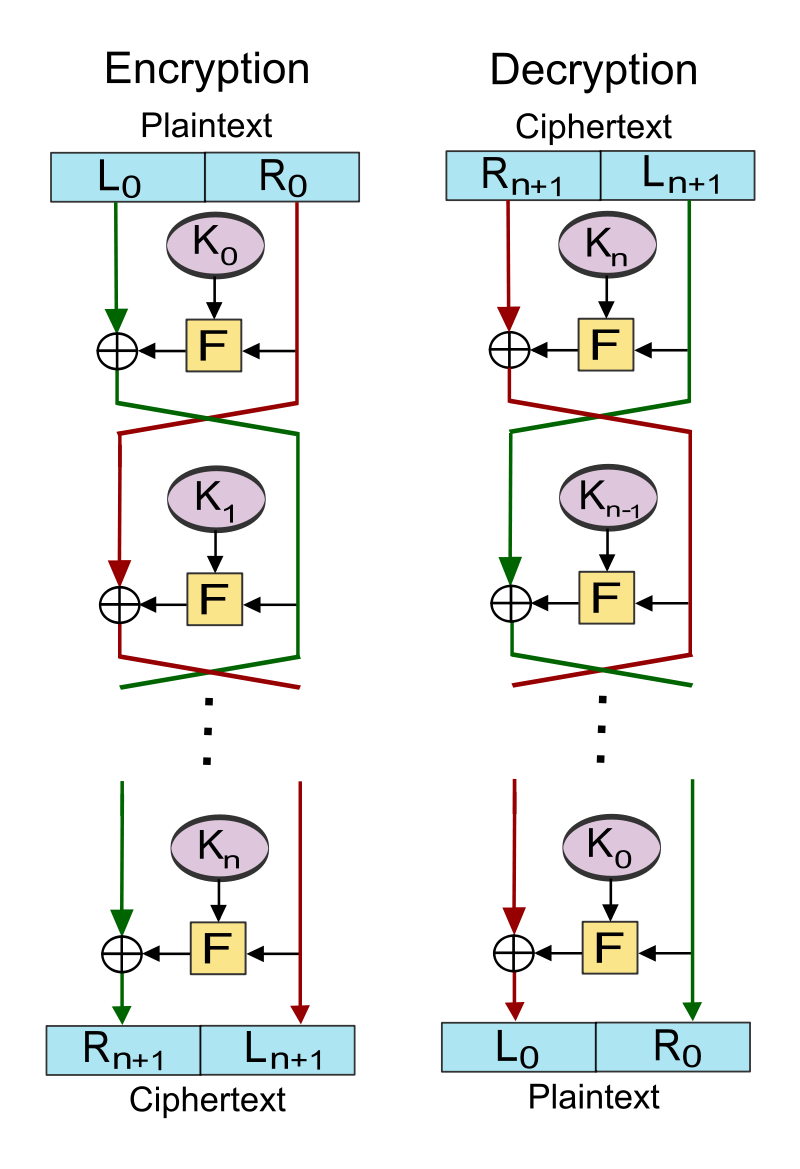## Data Encryption Standard

• A symmetric-key algorithm standardized in 1977
• Incorporates 16 Feistel Cipher rounds
• Has a block size of 64-bits
• Has a key size of 56-bits
• Purposely neutered by the NSA to make brute-force attacks easier

## How the Data Encryption Standard Works

• Expansion: Function that takes 32-bit block increased to 48-bits
• Key mixing: XOR operation with the subkey
• Substitution: Lookup table
• Permutation: Bit shuffling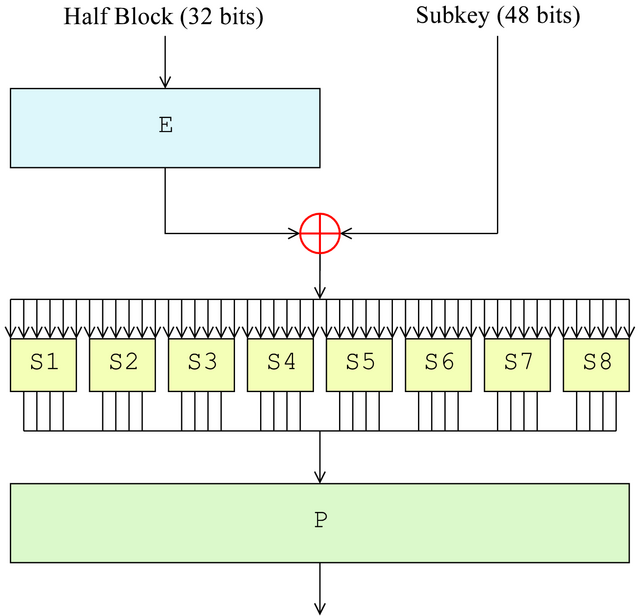## Triple DES (3DES)

• Published in 1981
• Incorporates 48 Feistel Cipher rounds
• Has a block size of 64-bits
• Has a key size of 168-bits (three 56-bit keys)
• Officially deprecated by NIST at the end of 2023• Published in 1998 by Joan Daemen and Vincent Rijmen under the name Rijndael
• Selected by NIST in 2001 to replace DES
Key length (bits) Block size (bits) # of Rounds
AES-128 128 128 10
AES-192 192 128 12
AES-256 256 128 14

## How AES works (1 of 2)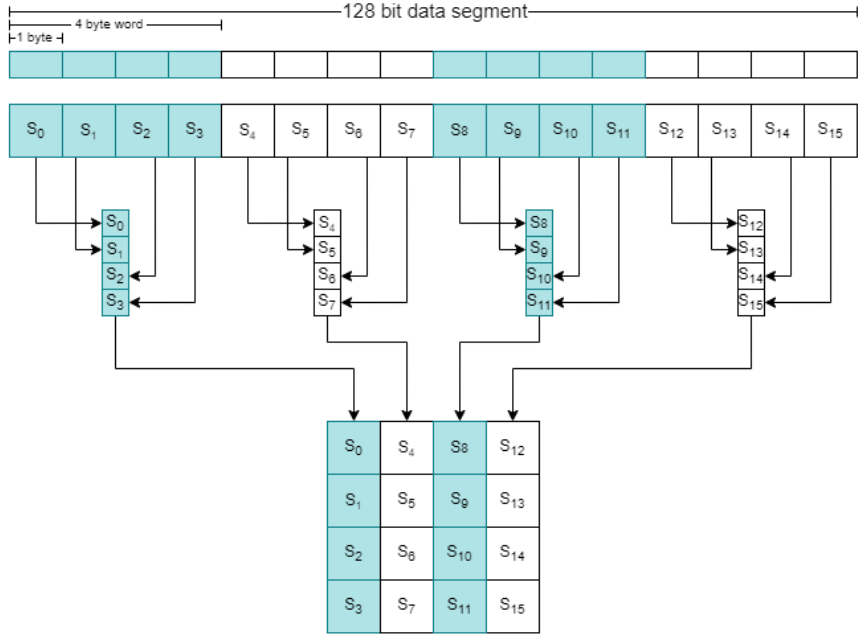## How AES works (2 of 2)

• SubBytes
• ShiftRows
• MixColumns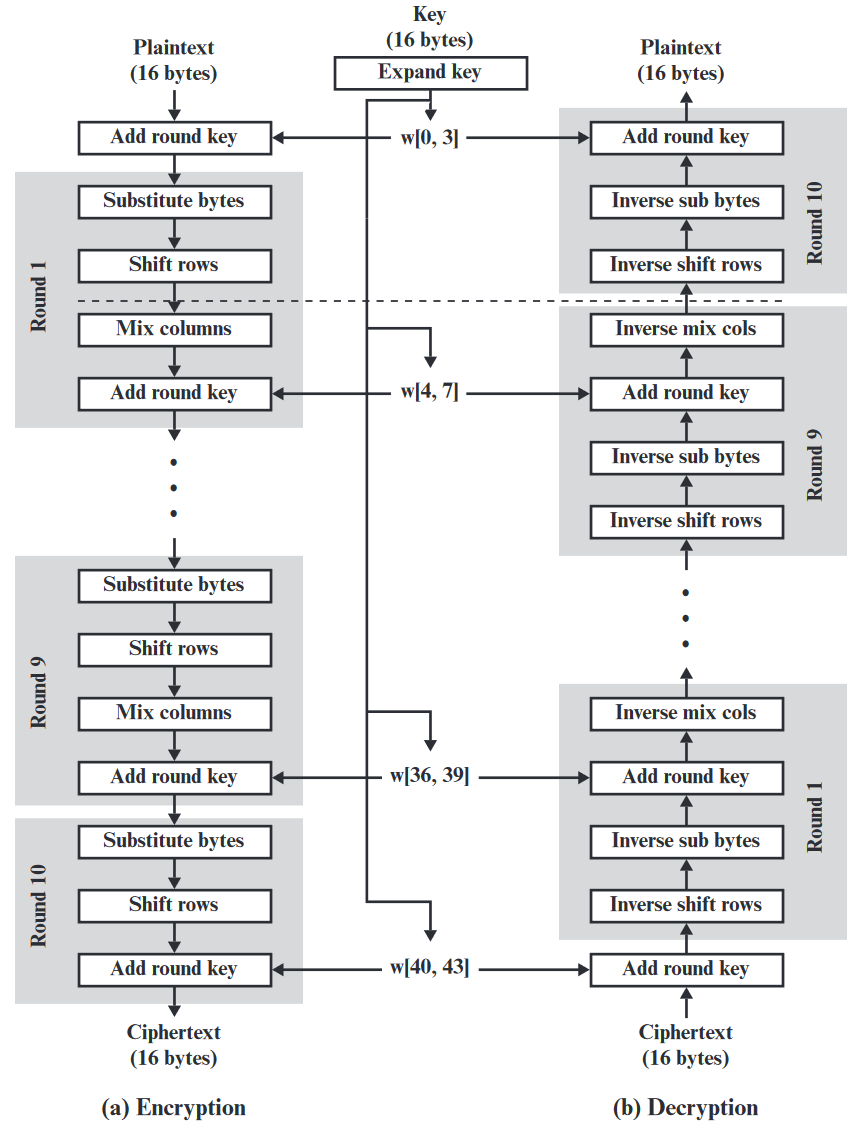## How AES works - SubBytes

• Byte-wise table lookup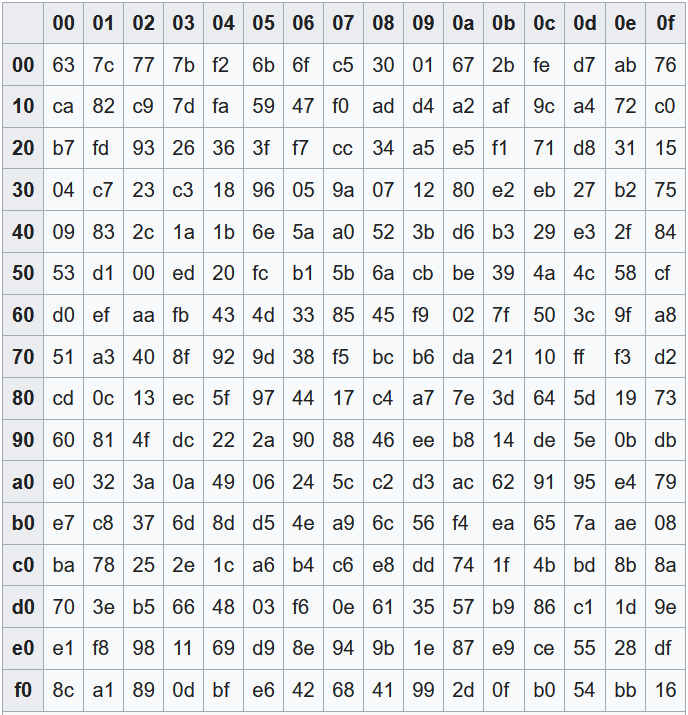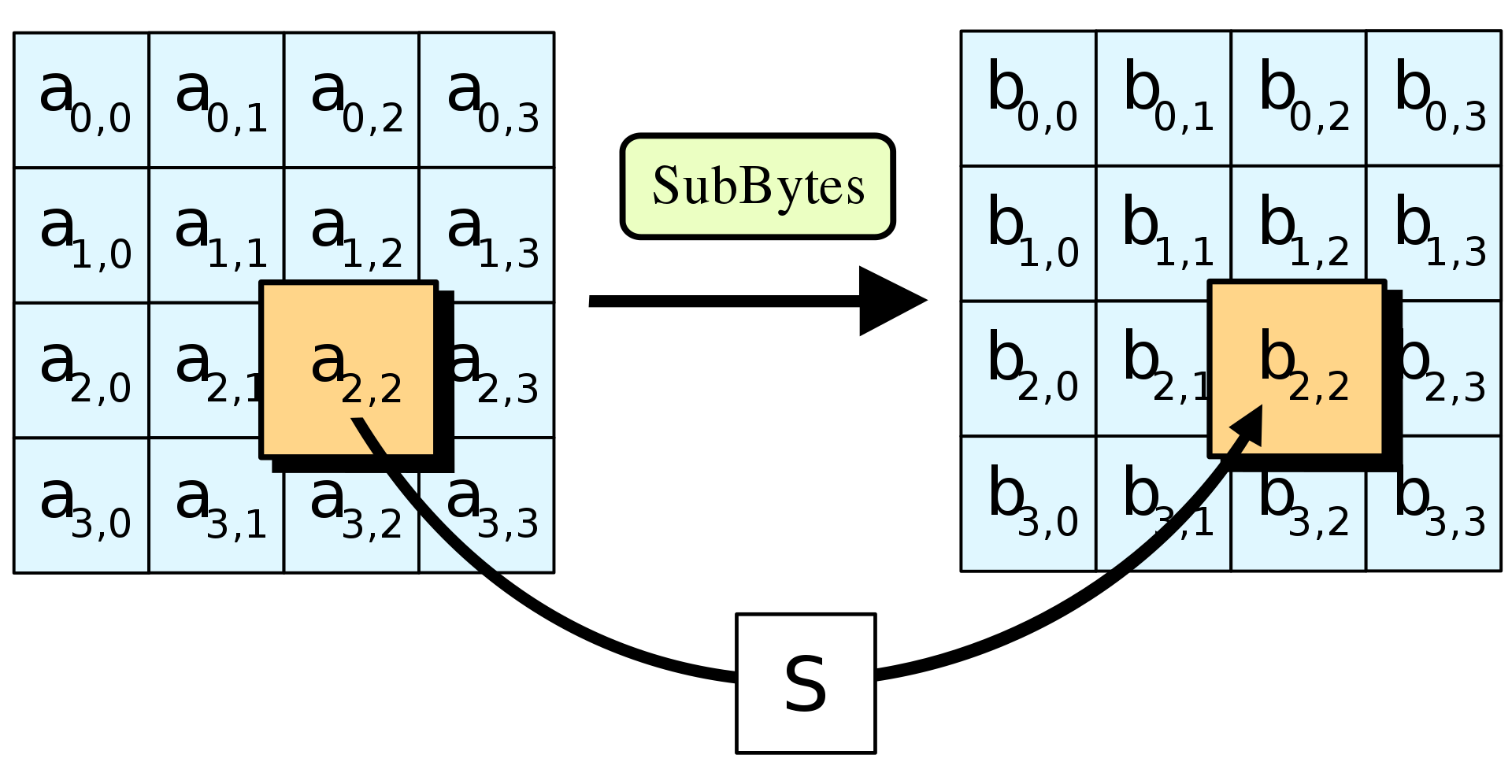## How AES works - ShiftRows## How AES works - MixColumns

• Shuffles bits using special matrix multiplication
• Uses polynomials, XOR, and modulo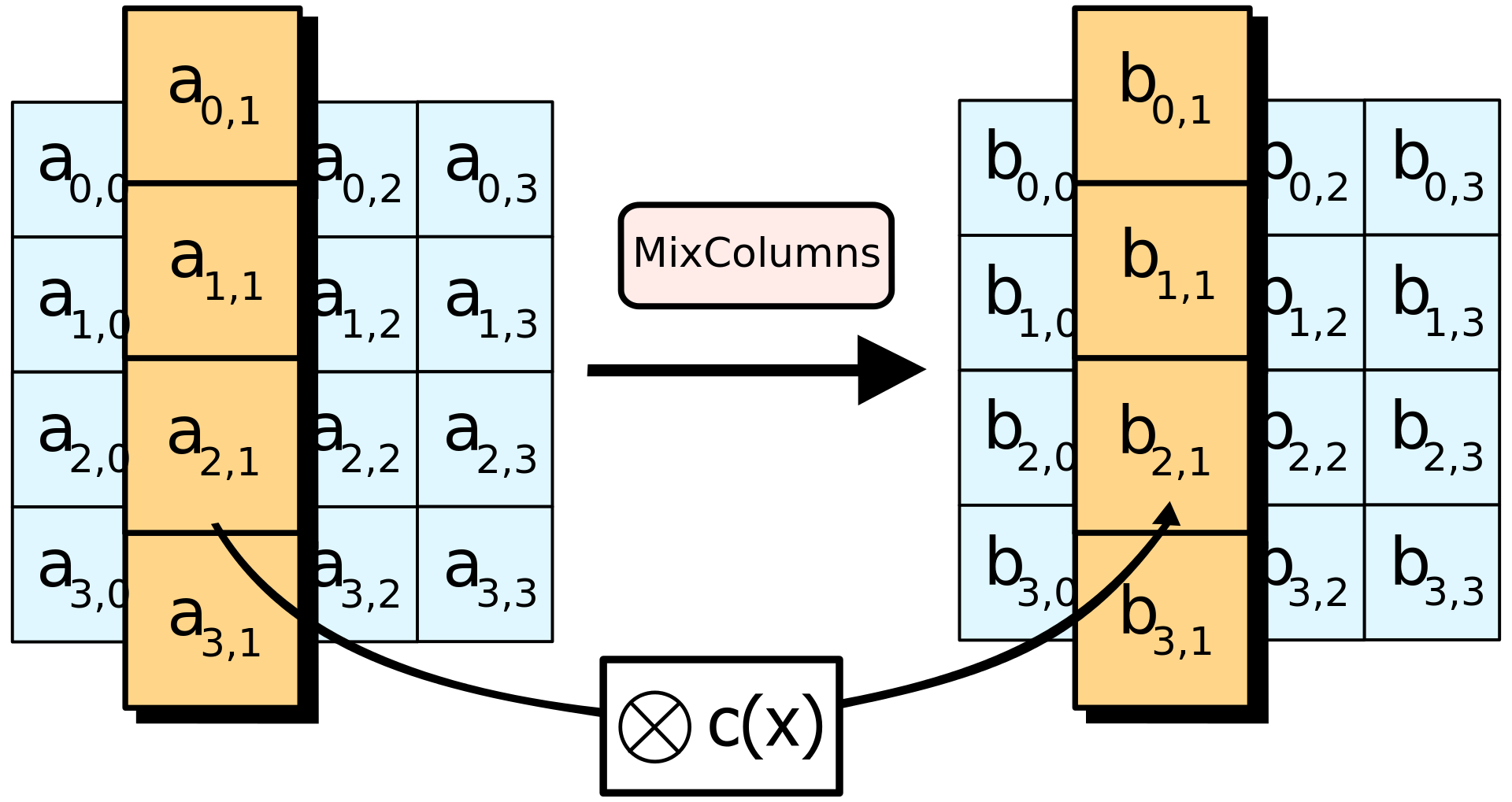## How AES works - AddRoundKey## Asymmetric Encryption## Diffie Hellman key exchange (DH)

• An asymmetric-key protocol conceived by Ralph Merkle that was published by Whitfield Diffie and Martin Hellman in 1976.

• Previously been discovered by a British intelligence agency in 1969.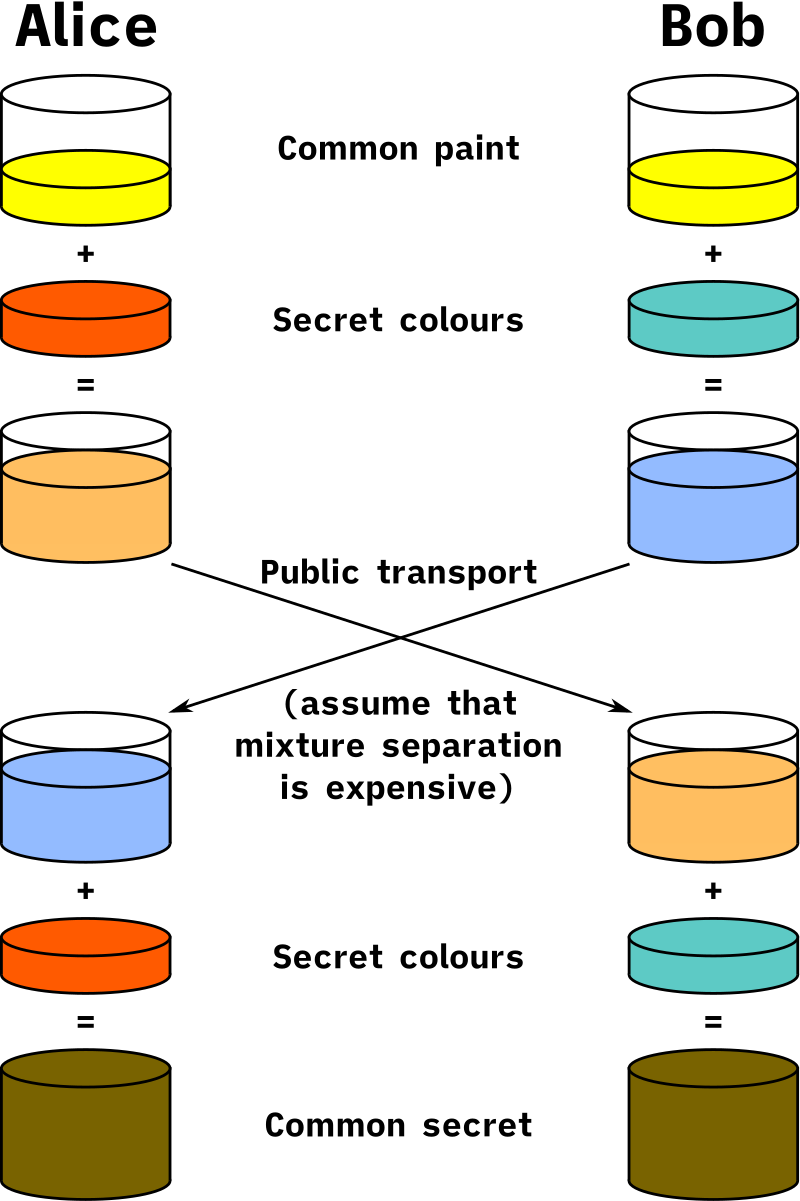## How DH Works (1 of 2)

• Alice and Bob publicly agree to use the modulus p=23 and base g=5
Alice Bob
α = 4 β = 3
A = gᵃ mod p B = gᵇ mod p
A = 5⁴ mod 23 = 625 mod 23 = 4 B = 5³ mod 23 = 125 mod 23 = 10
sᵃ = Bᵃ mod p sᵇ = Aᵇ mod p
sᵃ = 10⁴ mod 23 = 10000 mod 23 = 18 sᵇ = 4³ mod 23 = 64 mod 23 = 18

## How DH Works (2 of 2)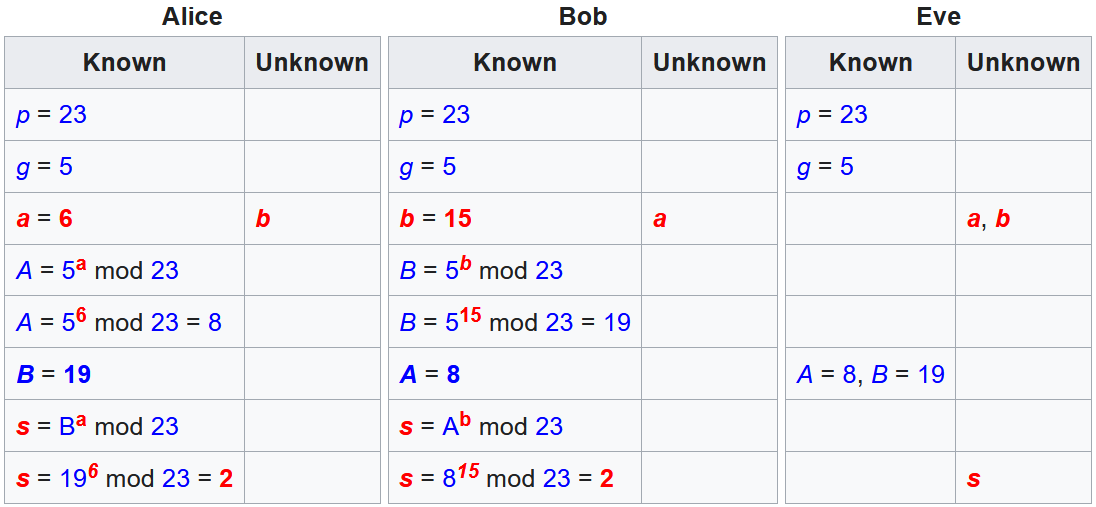• An asymmetric-key algorithm published in 1977 by Ron Rivest, Adi Shamir, and Leonard Adleman
• Relies on the difficulty of factoring the product of two large prime numbers
• Common key lengths in RSA
• 1024, 2048, 3072, 7680, 15360

## How RSA Works (1 of 2)

• p, q : chosen random prime integers (private)
• n : product of p and q, modulus for the public/private keys (public)
• Φ(n) : (Phi of n) Euler totient
• e : chosen integer that is (public):
• greater than one and less than the totient
• co-prime to the totient
• shares no common factors with the totient
• d : calculated multiplicative inverse of e mod the totient (private)

## How RSA Works (2 of 2)

• Public key

• {e, n}
• Private key

• {d, n}## RSA Example (1 of 3)

• p = 5, q = 7

• n = 5 × 7 = 35

• Φ(n) = (5 - 1) × (7 - 1) = 4 × 6 = 24

• e = 1 < 5 < 24

• can check if co-prime using Euclid’s Algorithm (GCD)
• d = 5⁻¹ mod 24 = 5

• can solve this using Extended Euclid’s Algorithm (XGCD)

## RSA Example (2 of 3)

• Public Key: {5, 35}

• plaintext (M): 23

• ciphertext (C) = Mᵉ mod n
• C = 23⁵ mod 35 = 6436343 mod 35 = 18

## RSA Example (3 of 3)

• Private Key: {5, 35}

• ciphertext (C): 18

• cleartext (M) = Cᵈ mod n
• M = 18⁵ mod 35 = 1889568 mod 35 = 23

## Elliptic-Curve Cryptography (ECC)

• An asymmetric-key cryptography approach independently suggested by Neal Koblitz and Victor S. Miller in 1985.
• Relies on the discrete logarithm problem over the elliptic curve y² = x³ + ax + b
• Elliptic-curve calculations are more computationally demanding allowing keys to be smaller than the equivalent RSA counterparts

## How ECC Works (1 of 4)

• Publicly agreed on curve (a=2, b=2, q=17):
• y² = x³ + ax + b
• y² = x³ + 2x + 2
• Publicly agreed on generator (G)
• G=(5,1)
• Publicly agreed order of G (n)
• n = 19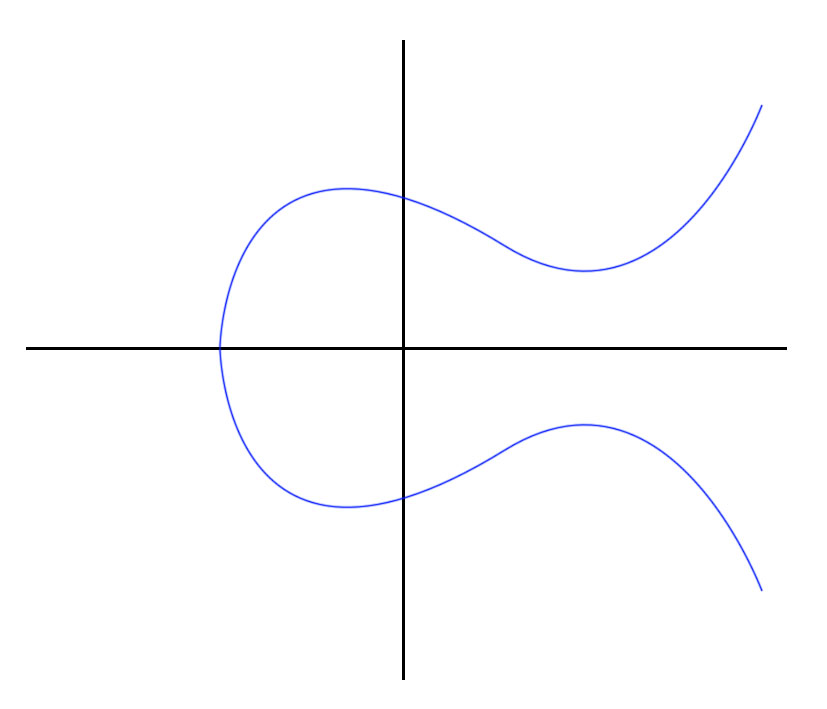## How ECC Works (2 of 4)

• G=(5,1)
Alice Bob
α = 3 β = 9
Α = 3G Β = 9G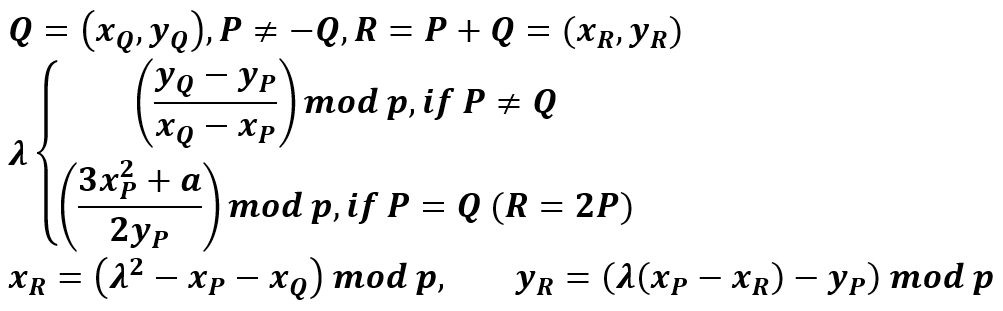## How ECC Works (3 of 4)

• Alice (3G)

• G + G = 2G
• 2G + G = 3G (10,6)
• Bob (9G)

• G + G = 2G
• 2G + 2G = 4G
• 4G + 4G = 8G
• 8G + G = 9G (7,6)## How ECC Works (4 of 4)

Alice Bob
Α = 3G = (10,6) Β = 9G = (7,6)
αΒ = 3Β = 3(9G) = 27G = 8G = (13,7) βΑ = 9Α = 9(3G) = 27G = 8G = (13,7)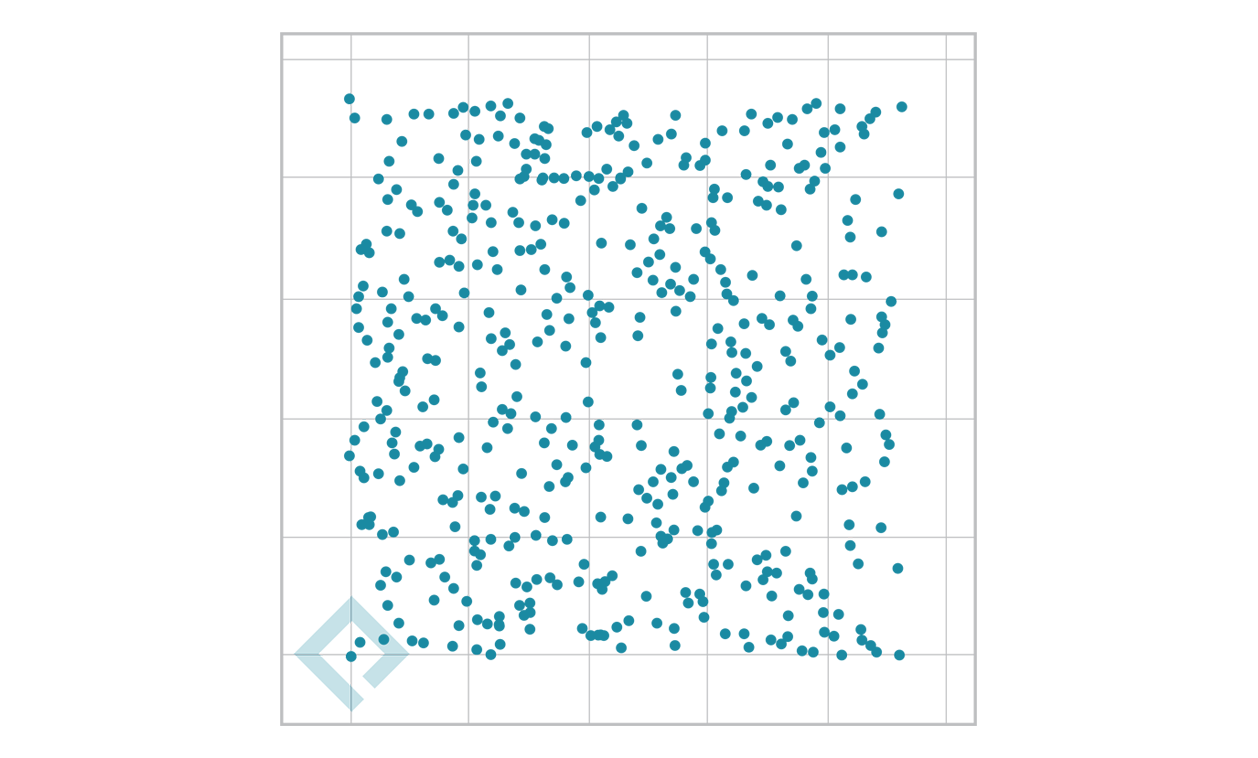## Comparison of Symmetric and Asymmetric Algorithms

Security Bits Symmetric Encryption Algorithm RSA ECC
80   1024 160
112 3DES 2048 224
128 AES-128 3072 256
192 AES-192 7680 384
256 AES-256 15360 512

## Symmetric Encryption Quantum Threats

• Grover’s Algorithm

• A quantum brute-forcing algorithm that is effective against AES

• Takes AES from O(N) to O(√N)

• 2¹²⁸ → 2⁶⁴

• 2¹⁹² → 2⁹⁶

• 2²⁵⁶ → 2¹²⁸

## Asymmetric Encryption Quantum Threats

• Shor’s Algorithm

• A quantum algorithm for finding prime factors of an integer (DH, RSA) and discrete logarithms (ECC)

## Hashing• SHA-256

• ef92b778bafe771e89245b89ecbc08a44a4e166c06659911881f383d4473e94f

• 9C9B913EB1B6254F4737CE947EFD16F16E916F9D6EE5C1102A2002E48D4C88BD

• fc91e3c64406e6afb456303d93ecb7c22b7f7ddb7f89d7e3ca52de8c10c16525

## Storing Hashed Passwords - LAN Manager

• Morpheus:1010:B902F044A44585DF93E28745B8BF4BA6:D5D630DA69EBD8AB040D77BE7BB046DD:::

• Converted to uppercase
• Unused space filled with null bytes
• Split in half with parity character added (two 8-byte segments)
• Both halves are encrypted with DES and concatenated together

## Storing Hashed Passwords - New Technology LAN Manager

• RC4 encryption

## Storing Hashed Passwords - sha512crypt

• Morpheus:\$6\$ykumbyCz\$kb1zUhrB3wROIN1uoI94gfmAMjlTeeVhKkEtEZHYeIFIqD6CBiAQAlFB9uqYHHVO1doCNJbV56tC4gJi/L0ng0:1001:1001::/home/morpheus:/bin/sh

## `man 5 crypt` - sha512crypt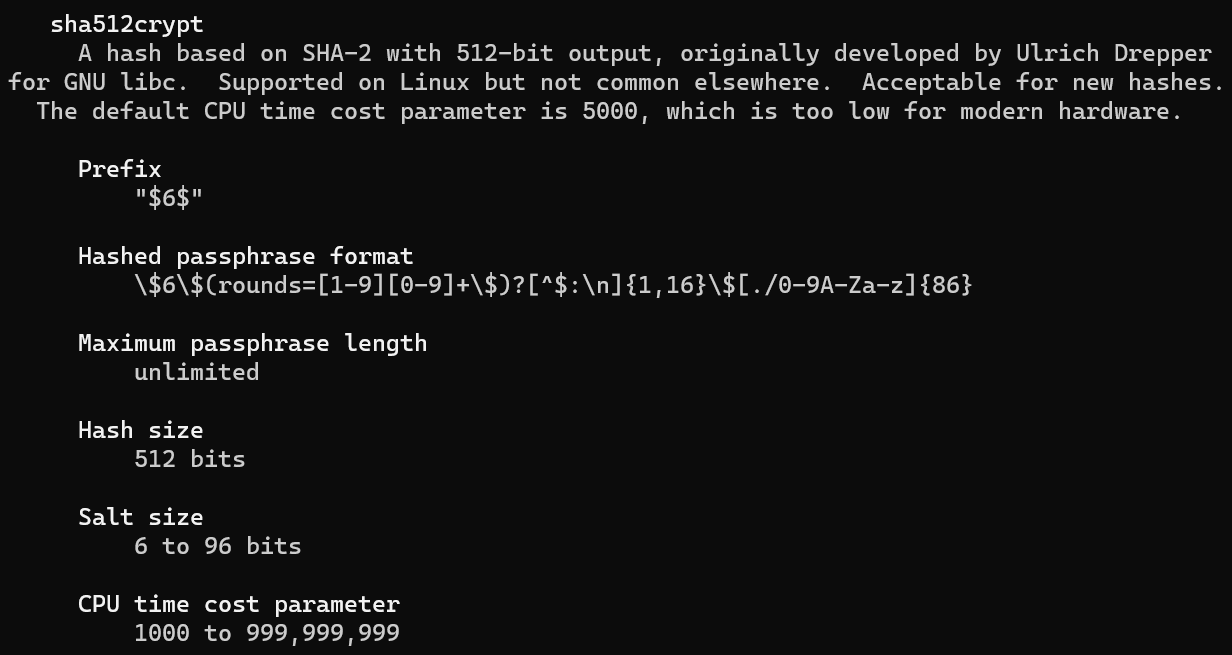## `man 5 crypt` - yescrypt